2020年末，OneFlow 发布了《OneFlow 性能优化分享：如何实现一个高效的 Softmax CUDA kernel？》，其中介绍了OneFlow深度优化后的Softmax，尤其对很多框架没有考虑的 half 类型做了充分优化，使得性能大幅超过了 cuDNN 的实现。

1 OneFlow 性能优化后的测试结果

OneFlow 优化后的 LayerNorm 分别与 NVIDIA Apex、PyTorch 做了性能对比，测试结果显示，OneFlow LayerNorm 有明显的性能优势。

NVIDIA Apex 中实现了高效的 fused LayerNorm Kernel 来扩展 PyTorch 算子，我们对 OneFlow 优化后的 LayerNorm Kernel 和 NVIDIA Apex 进行了对比测试，测试结果如下：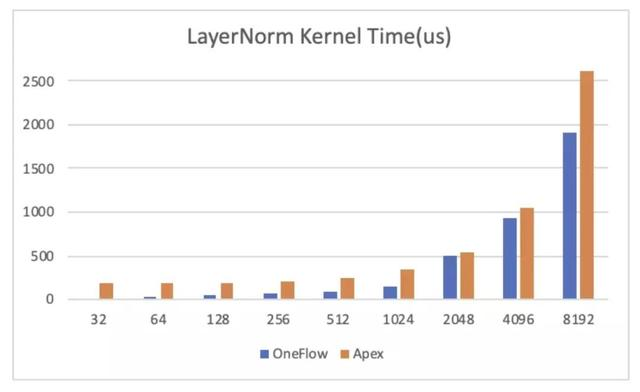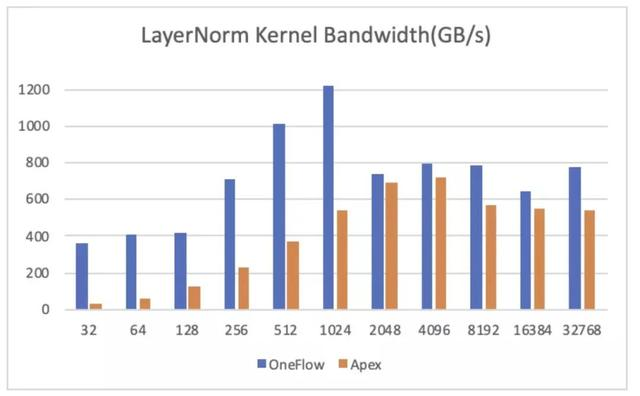PyTorch 的 LayerNorm 暂时不支持 half 类型，因此我们用 float类型做了一组对照，需要注意的是PyTorch中LayerNorm是分两个CUDA Kernel(RowwiseMomentsCUDAKernel和LayerNormForwardCUDAKernel)做的，所以看起来性能比较差。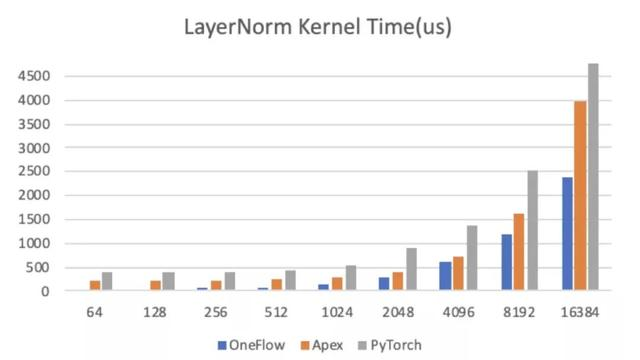LayerNorm 性能优化

LayerNorm 是语言模型中常用的操作之一，其 CUDA Kernel 实现的高效性会影响很多网络最终的训练速度，Softmax 的优化方法也适用于 LayerNorm，LayerNorm 的数据也可以表示为 (num_rows, num_cols)，计算过程中对每一行的元素做 Reduce 操作求均值方差。因此我们使用了和 Softmax 同样的优化方法来优化 LayerNorm 操作，本文以 LayerNorm 前向计算为例进行介绍。

LayerNorm 计算方法

`torch.nn.LayerNorm(normalized_shape, eps=1e-05, elementwise_affine=True, device=None, dtype=None)`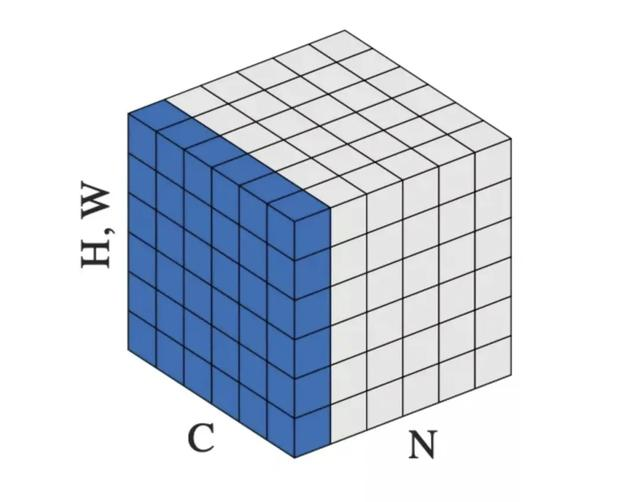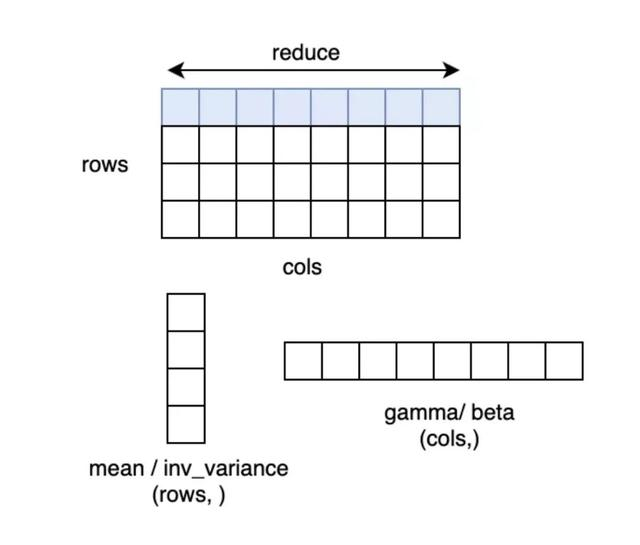LayerNorm 中求方差的方法

1.two-pass方法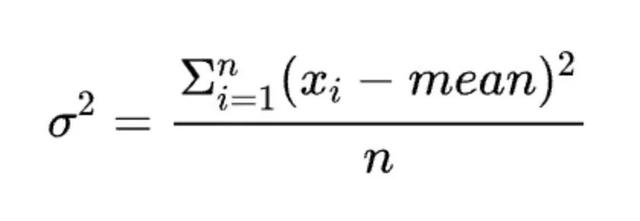two-pass 是指这种方法需要遍历两遍数据，第一遍累加 x 得到均值，第二遍用上面公式计算得到方差。这种方法在 n 比较小时仍然是数值稳定的。

2.naive方法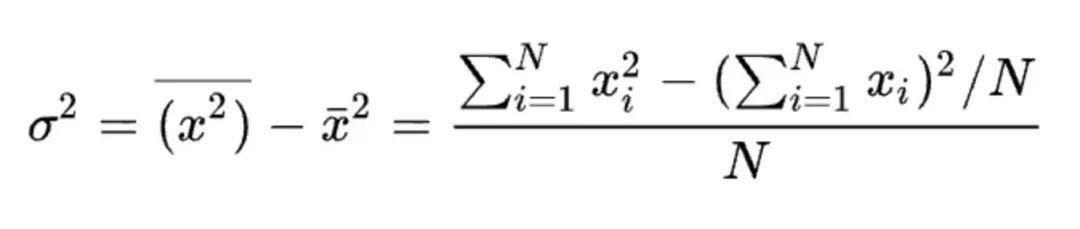3.Welford 算法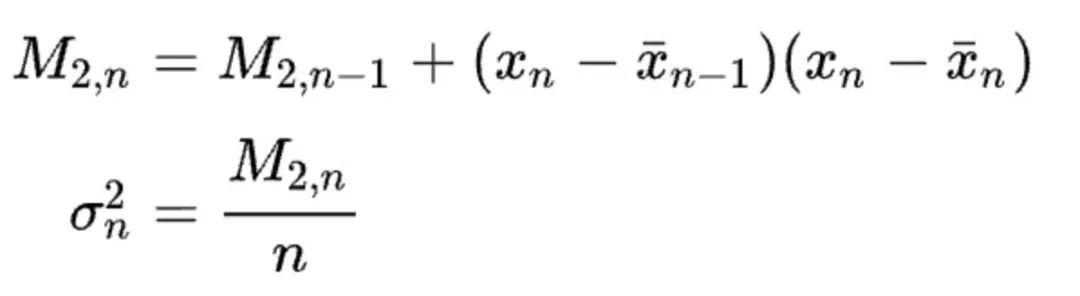Welford 算法也是一种 single pass 方法，且数值稳定性很好，因此现在很多框架都采用这种方法。本文的代码中采用的也是 Welford 方法。

OneFlow 深度优化 LayerNorm CUDA Kernel 的技巧

1.num_cols <= 1024 的情况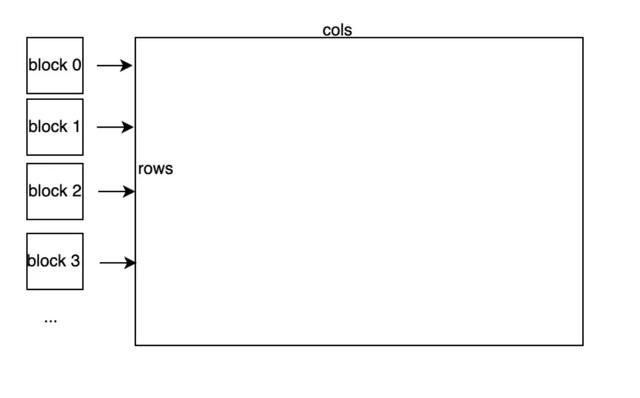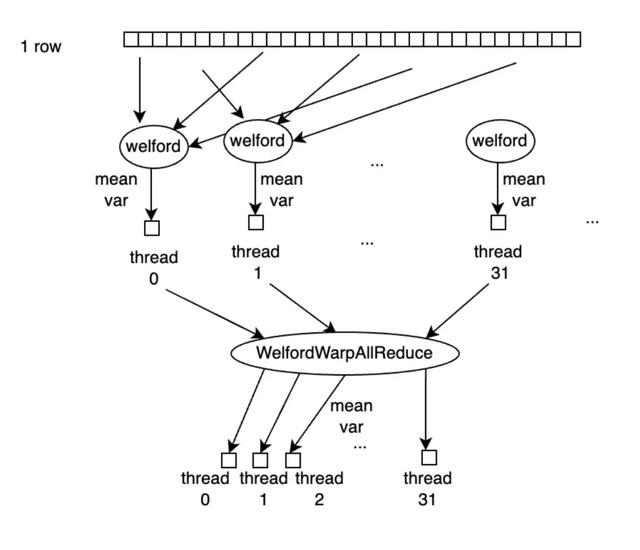WelfordWarpAllReduce 由 WelfordWarpReduce 和 Broadcast 操作完成，WelfordWarpReduce 借助 Warp 级别同步原语_\_shfl\_down\_sync 实现，Broadcast操作借助 _\_shfl\_sync 实现，代码如下：

`template<typename T, int thread_group_width = kWarpSize>__inline__ __device__ void WelfordWarpReduce(T thread_mean, T thread_m2, T thread_count, T* mean, T* m2, T* count) { *mean = thread_mean; *m2 = thread_m2; *count = thread_count; for (int mask = thread_group_width / 2; mask > 0; mask /= 2) { T b_mean = __shfl_down_sync(0xffffffff, *mean, mask); T b_m2 = __shfl_down_sync(0xffffffff, *m2, mask); T b_count = __shfl_down_sync(0xffffffff, *count, mask); WelfordCombine(b_mean, b_m2, b_count, mean, m2, count); }}template<typename T, int thread_group_width = kWarpSize>__inline__ __device__ void WelfordWarpAllReduce(T thread_mean, T thread_m2, T thread_count, T* mean, T* m2, T* count) { WelfordWarpReduce<T, thread_group_width>(thread_mean, thread_m2, thread_count, mean, m2, count); *mean = __shfl_sync(0xffffffff, *mean, 0, thread_group_width); *m2 = __shfl_sync(0xffffffff, *m2, 0, thread_group_width); *count = __shfl_sync(0xffffffff, *count, 0, thread_group_width);`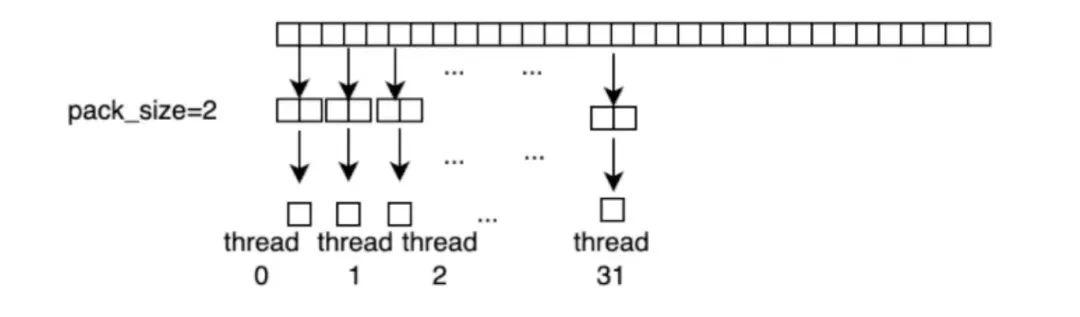`template\<typename T, int N> union Pack \{ PackType\<T, N> storage; T elem\[N\]; \}; `

LayerNormWarpImpl Kernel 代码如下：

`template<typename LOAD, typename STORE, typename ComputeType, int pack_size, int cols_per_thread, int thread_group_width, int rows_per_access, bool padding>__global__ void LayerNormWarpImpl(LOAD load, STORE store, const int64_t rows, const int64_t cols, const double epsilon, ComputeType* mean, ComputeType* inv_variance) { static_assert(cols_per_thread % pack_size == 0, ""); static_assert(thread_group_width <= kWarpSize, ""); static_assert(kWarpSize % thread_group_width == 0, ""); constexpr int num_packs = cols_per_thread / pack_size; assert(cols <= cols_per_thread * thread_group_width); ComputeType buf[rows_per_access][cols_per_thread]; const int64_t global_thread_group_id = blockIdx.x * blockDim.y + threadIdx.y; const int64_t num_global_thread_group = gridDim.x * blockDim.y; const int64_t lane_id = threadIdx.x; for (int64_t row = global_thread_group_id * rows_per_access; row < rows; row += num_global_thread_group * rows_per_access) { ComputeType thread_mean[rows_per_access]; ComputeType thread_m2[rows_per_access]; ComputeType thread_count[rows_per_access];#pragma unroll for (int row_id = 0; row_id < rows_per_access; ++row_id) { thread_mean[row_id] = 0; thread_m2[row_id] = 0; thread_count[row_id] = 0; ComputeType* row_buf = buf[row_id];#pragma unroll for (int pack_id = 0; pack_id < num_packs; ++pack_id) { const int col = (pack_id * thread_group_width + lane_id) * pack_size; const int pack_offset = pack_id * pack_size; if (!padding || col < cols) { load.template load<pack_size>(row_buf + pack_offset, row + row_id, col);#pragma unroll for (int i = 0; i < pack_size; ++i) { WelfordCombine(row_buf[pack_offset + i], thread_mean + row_id, thread_m2 + row_id, thread_count + row_id); } } else {#pragma unroll for (int i = 0; i < pack_size; ++i) { row_buf[pack_offset + i] = 0; } } } } ComputeType warp_mean[rows_per_access]; ComputeType warp_m2[rows_per_access]; ComputeType warp_count[rows_per_access];#pragma unroll for (int row_id = 0; row_id < rows_per_access; ++row_id) { int global_row_id = row + row_id; ComputeType* row_buf = buf[row_id]; WelfordWarpAllReduce<ComputeType, thread_group_width>( thread_mean[row_id], thread_m2[row_id], thread_count[row_id], warp_mean + row_id, warp_m2 + row_id, warp_count + row_id); ComputeType row_mean = warp_mean[row_id]; ComputeType row_variance = max(Div(warp_m2[row_id], warp_count[row_id]), static_cast<ComputeType>(0.0)); ComputeType row_inv_var = Rsqrt(row_variance + static_cast<ComputeType>(epsilon)); if (lane_id == 0) { mean[global_row_id] = row_mean; inv_variance[global_row_id] = row_inv_var; }#pragma unroll for (int i = 0; i < cols_per_thread; ++i) { row_buf[i] = (row_buf[i] - row_mean) * row_inv_var; }#pragma unroll for (int i = 0; i < num_packs; ++i) { const int col = (i * thread_group_width + lane_id) * pack_size; if (!padding || col < cols) { store.template store<pack_size>(row_buf + i * pack_size, global_row_id, col); } } } }}`

LayerNormWarpImpl 的实现的模板参数的意义分别如下：

2.num\_cols > 1024 的情况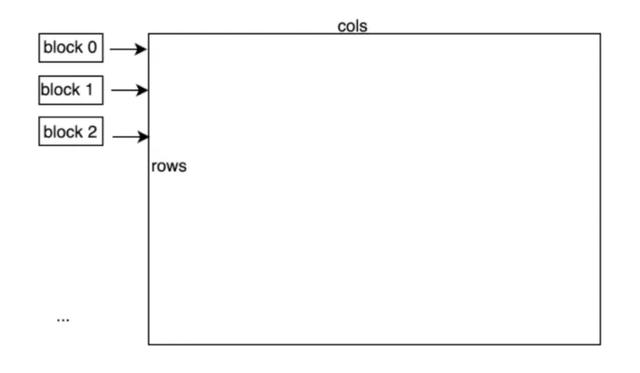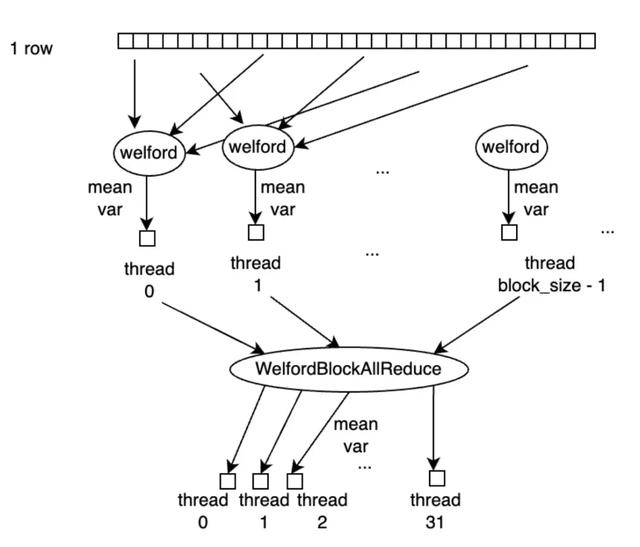WelfordBlockAllReduce 是借助 WelfordWarpReduce 操作完成的，具体逻辑是，一个 Block 中最多有32个 Warp，对所有的 Warp 先执行一次 WelfordWarpReduce，执行完后，每个 warp 中的第一个线程，即 lane\_id=0 的线程上得到当前 WelfordWarpReduce 的结果，再将每个 Warp 的第一个线程的结果拷贝到一块 Shared Memory buffer 中，再用第一个 Warp 的32个线程执行一次 WelfordWarpReduce，此时第一个 Warp 中的 lane\_id=0 的线程上得到的就是 block 中所有线程reduce 的结果。再借助 Shared Memory，将该结果 broadcast 到 block 中的所有线程上，即完成了 WelfordBlockAllReduce 的操作。

LayerNormBlockSMemImpl Kernel的代码如下：

`template\<typename LOAD, typename STORE, typename ComputeType, int pack\_size, int block\_size> \_\_global\_\_ void LayerNormBlockSMemImpl\(LOAD load, STORE store, const int64\_t rows, const int64\_t cols, const double epsilon, ComputeType\* mean, ComputeType\* inv\_variance\) \{ extern \_\_shared\_\_ \_\_align\_\_\(sizeof\(double\)\) unsigned char shared\_buf\[\]; auto\* buf = reinterpret\_cast\<ComputeType\*>\(shared\_buf\); const int tid = threadIdx.x; assert\(cols \% pack\_size == 0\); const int num\_packs = cols / pack\_size; for \(int64\_t row = blockIdx.x; row \< rows; row += gridDim.x\) \{ ComputeType thread\_mean = 0; ComputeType thread\_m2 = 0; ComputeType thread\_count = 0; for \(int pack\_id = tid; pack\_id \< num\_packs; pack\_id += block\_size\) \{ ComputeType pack\[pack\_size\]; load.template load\<pack\_size>\(pack, row, pack\_id \* pack\_size\); #pragma unroll for \(int i = 0; i \< pack\_size; ++i\) \{ buf\[i \* num\_packs + pack\_id\] = pack\[i\]; WelfordCombine\(pack\[i\], \&thread\_mean, \&thread\_m2, \&thread\_count\); \} \} ComputeType row\_mean = 0; ComputeType row\_m2 = 0; ComputeType row\_count = 0; WelfordBlockAllReduce\<ComputeType>\(thread\_mean, thread\_m2, thread\_count, \&row\_mean, \&row\_m2, \&row\_count\); ComputeType row\_variance = max\(Div\(row\_m2, row\_count\), static\_cast\<ComputeType>\(0.0\)\); ComputeType row\_inv\_var = Rsqrt\(row\_variance + static\_cast\<ComputeType>\(epsilon\)\); if \(threadIdx.x == 0\) \{ mean\[row\] = row\_mean; inv\_variance\[row\] = row\_inv\_var; \} for \(int pack\_id = tid; pack\_id \< num\_packs; pack\_id += block\_size\) \{ ComputeType pack\[pack\_size\]; #pragma unroll for \(int i = 0; i \< pack\_size; ++i\) \{ pack\[i\] = \(buf\[i \* num\_packs + pack\_id\] \- row\_mean\) \* row\_inv\_var; \} store.template store\<pack\_size>\(pack, row, pack\_id \* pack\_size\); \} \} \} `

3.num_cols 较大时，不使用 Shared Memory 的情

LayerNormBlockUncachedImpl 代码如下：

`template\<typename LOAD, typename STORE, typename ComputeType, int pack\_size, int block\_size> \_\_global\_\_ void LayerNormBlockUncachedImpl\(LOAD load, STORE store, const int64\_t rows, const int64\_t cols, const double epsilon, ComputeType\* mean, ComputeType\* inv\_variance\) \{ const int tid = threadIdx.x; assert\(cols \% pack\_size == 0\); const int num\_packs = cols / pack\_size; for \(int64\_t row = blockIdx.x; row \< rows; row += gridDim.x\) \{ ComputeType thread\_mean = 0; ComputeType thread\_m2 = 0; ComputeType thread\_count = 0; for \(int pack\_id = tid; pack\_id \< num\_packs; pack\_id += block\_size\) \{ ComputeType pack\[pack\_size\]; load.template load\<pack\_size>\(pack, row, pack\_id \* pack\_size\); #pragma unroll for \(int i = 0; i \< pack\_size; ++i\) \{ WelfordCombine\(pack\[i\], \&thread\_mean, \&thread\_m2, \&thread\_count\); \} \} ComputeType row\_mean = 0; ComputeType row\_m2 = 0; ComputeType row\_count = 0; WelfordBlockAllReduce\<ComputeType>\(thread\_mean, thread\_m2, thread\_count, \&row\_mean, \&row\_m2, \&row\_count\); ComputeType row\_variance = max\(Div\(row\_m2, row\_count\), static\_cast\<ComputeType>\(0.0\)\); ComputeType row\_inv\_var = Rsqrt\(row\_variance + static\_cast\<ComputeType>\(epsilon\)\); if \(threadIdx.x == 0\) \{ mean\[row\] = row\_mean; inv\_variance\[row\] = row\_inv\_var; \} for \(int pack\_id = tid; pack\_id \< num\_packs; pack\_id += block\_size\) \{ ComputeType pack\[pack\_size\]; const int pack\_offset = pack\_id \* pack\_size; load.template load\<pack\_size>\(pack, row, pack\_offset\); #pragma unroll for \(int i = 0; i \< pack\_size; ++i\) \{ pack\[i\] = \(pack\[i\] \- row\_mean\) \* row\_inv\_var; \} store.template store\<pack\_size>\(pack, row, pack\_offset\); \} \} \}`

3 OneFlow Softmax 库

` oneflow::cuda::softmax::DirectLoad\<half, float\> load\(in, cols\); oneflow::cuda::softmax::DirectStore\<float, half> store\(out, cols\); oneflow::cuda::softmax::DispatchSoftmax\<decltype\(load\), decltype\(store\), float\>\( cuda\_stream, load, store, rows, cols\); `